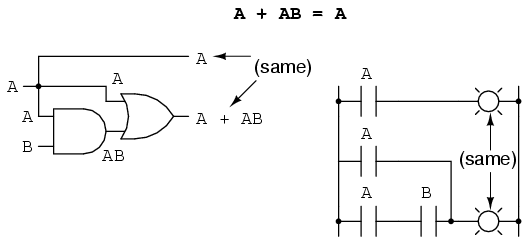# 7.5: Boolean Rules for Simplification

$$\newcommand{\vecs}{\overset { \rightharpoonup} {\mathbf{#1}} }$$ $$\newcommand{\vecd}{\overset{-\!-\!\rightharpoonup}{\vphantom{a}\smash {#1}}}$$$$\newcommand{\id}{\mathrm{id}}$$ $$\newcommand{\Span}{\mathrm{span}}$$ $$\newcommand{\kernel}{\mathrm{null}\,}$$ $$\newcommand{\range}{\mathrm{range}\,}$$ $$\newcommand{\RealPart}{\mathrm{Re}}$$ $$\newcommand{\ImaginaryPart}{\mathrm{Im}}$$ $$\newcommand{\Argument}{\mathrm{Arg}}$$ $$\newcommand{\norm}{\| #1 \|}$$ $$\newcommand{\inner}{\langle #1, #2 \rangle}$$ $$\newcommand{\Span}{\mathrm{span}}$$ $$\newcommand{\id}{\mathrm{id}}$$ $$\newcommand{\Span}{\mathrm{span}}$$ $$\newcommand{\kernel}{\mathrm{null}\,}$$ $$\newcommand{\range}{\mathrm{range}\,}$$ $$\newcommand{\RealPart}{\mathrm{Re}}$$ $$\newcommand{\ImaginaryPart}{\mathrm{Im}}$$ $$\newcommand{\Argument}{\mathrm{Arg}}$$ $$\newcommand{\norm}{\| #1 \|}$$ $$\newcommand{\inner}{\langle #1, #2 \rangle}$$ $$\newcommand{\Span}{\mathrm{span}}$$$$\newcommand{\AA}{\unicode[.8,0]{x212B}}$$

Boolean algebra finds its most practical use in the simplification of logic circuits. If we translate a logic circuit’s function into symbolic (Boolean) form, and apply certain algebraic rules to the resulting equation to reduce the number of terms and/or arithmetic operations, the simplified equation may be translated back into circuit form for a logic circuit performing the same function with fewer components. If equivalent function may be achieved with fewer components, the result will be increased reliability and decreased cost of manufacture.

To this end, there are several rules of Boolean algebra presented in this section for use in reducing expressions to their simplest forms. The identities and properties already reviewed in this chapter are very useful in Boolean simplification, and for the most part bear similarity to many identities and properties of “normal” algebra. However, the rules shown in this section are all unique to Boolean mathematics.This rule may be proven symbolically by factoring an “A” out of the two terms, then applying the rules of A + 1 = 1 and 1A = A to achieve the final result:Please note how the rule A + 1 = 1 was used to reduce the (B + 1) term to 1. When a rule like “A + 1 = 1” is expressed using the letter “A”, it doesn’t mean it only applies to expressions containing “A”. What the “A” stands for in a rule like A + 1 = 1 is any Boolean variable or collection of variables. This is perhaps the most difficult concept for new students to master in Boolean simplification: applying standardized identities, properties, and rules to expressions not in standard form.

For instance, the Boolean expression ABC + 1 also reduces to 1 by means of the “A + 1 = 1” identity. In this case, we recognize that the “A” term in the identity’s standard form can represent the entire “ABC” term in the original expression.

The next rule looks similar to the first one shown in this section, but is actually quite different and requires a more clever proof:Note how the last rule (A + AB = A) is used to “un-simplify” the first “A” term in the expression, changing the “A” into an “A + AB”. While this may seem like a backward step, it certainly helped to reduce the expression to something simpler! Sometimes in mathematics we must take “backward” steps to achieve the most elegant solution. Knowing when to take such a step and when not to is part of the art-form of algebra, just as a victory in a game of chess almost always requires calculated sacrifices.

Another rule involves the simplification of a product-of-sums expression:And, the corresponding proof:To summarize, here are the three new rules of Boolean simplification expounded in this section:This page titled 7.5: Boolean Rules for Simplification is shared under a GNU Free Documentation License 1.3 license and was authored, remixed, and/or curated by Tony R. Kuphaldt (All About Circuits) via source content that was edited to the style and standards of the LibreTexts platform; a detailed edit history is available upon request.